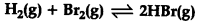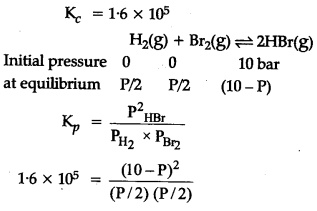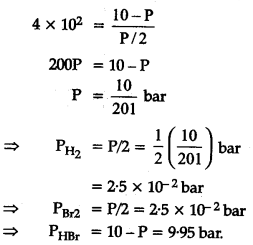# The equilibrium constant for the following reaction is 1.6 X 1\${{10}_{5}}\$ at 1024 K

The equilibrium constant for the following reaction is 1.6 X 1\${{10}_{5}}\$ at 1024 K.Find the equilibrium pressure of all gases if 10 bar of HBr is introduced into a sealed container at 1024 K ?Squaring on both sides